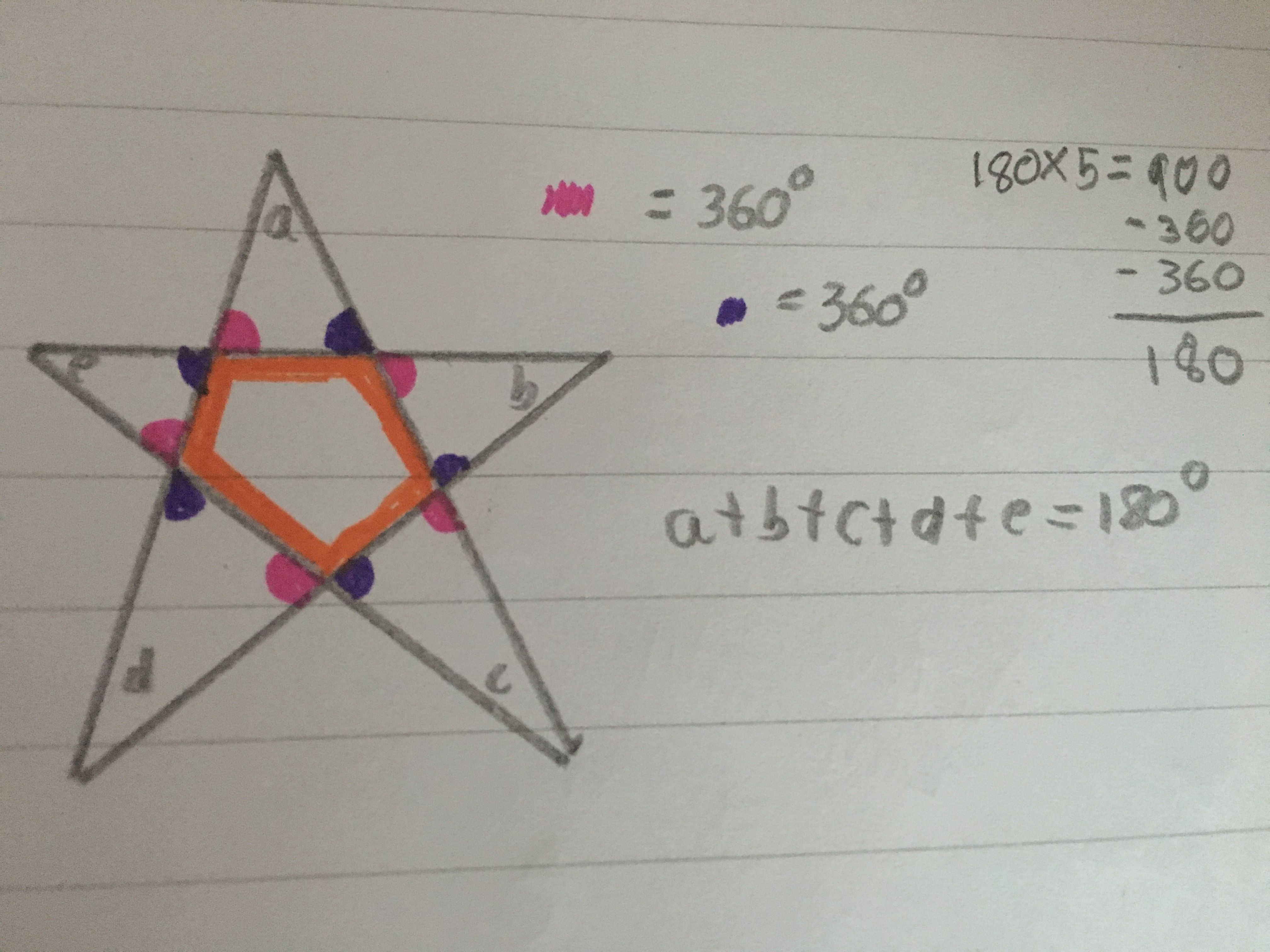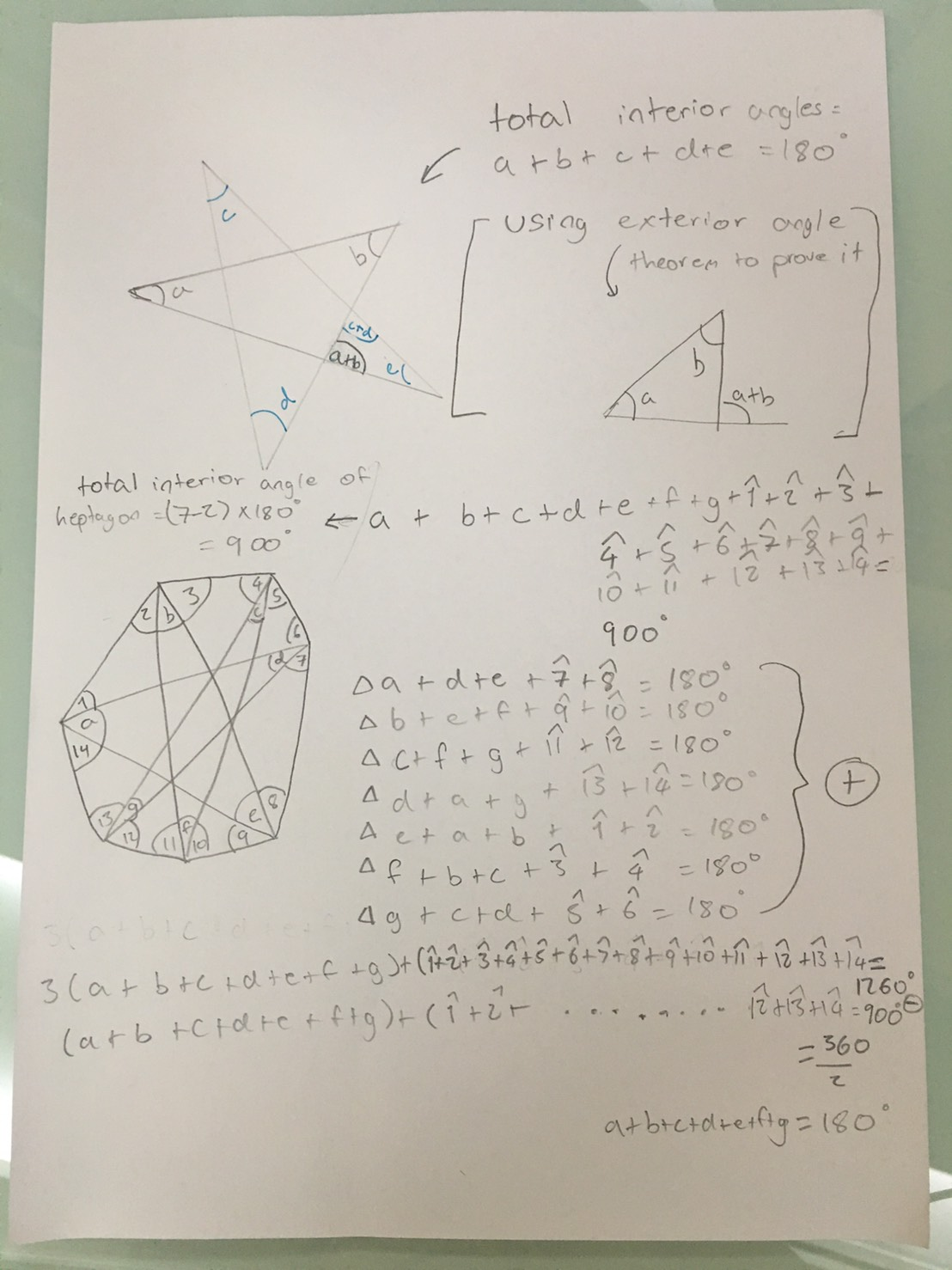#### You may also like### Getting an Angle

How can you make an angle of 60 degrees by folding a sheet of paper twice?### Arclets Explained

This article gives an wonderful insight into students working on the Arclets problem that first appeared in the Sept 2002 edition of the NRICH website.### Bow Tie

Show how this pentagonal tile can be used to tile the plane and describe the transformations which map this pentagon to its images in the tiling.

# Star Polygons

##### Age 11 to 14Challenge Level

Well done to Rose from Newstead Wood School in the United Kingdom and Melissa from Bangkok Patana School in Bangkok who sent us their solutions to this problem. They both used different methods prove this result.

Here is Rose's proof for the five-pointed star:

Notice there is a pentagon in the centre of the five point star. The exterior angles of this shape must add up to $360^\circ$. If the exterior angles are marked onto a diagram, each one is in a corner of an outer triangle, as shown below:Effectively, there are two sets of exterior angles on the central pentagon, depending on the direction in which they are marked. Mark these in (shown in pink and blue on the diagram above) and each outer triangle will have an angle drawn in two base corners. There are are five triangles within a five-pointed star. The angles in a triangle add up to $180^\circ$.  $180^\circ \times 5$ will give the total number of degrees in all the triangles of the star: $900^\circ$

Earlier, it was said exterior angles on the central pentagon had to equal $360^\circ$. Two sets of exterior angles have been marked on the shape. If both of these sets are subtracted from the total angles: $900^\circ - 360^\circ - 360^\circ = 180^\circ$ is left. This is the sum of the outer points of the star.

Melissa sent us the following proofs for the five- and seven-pointed stars: